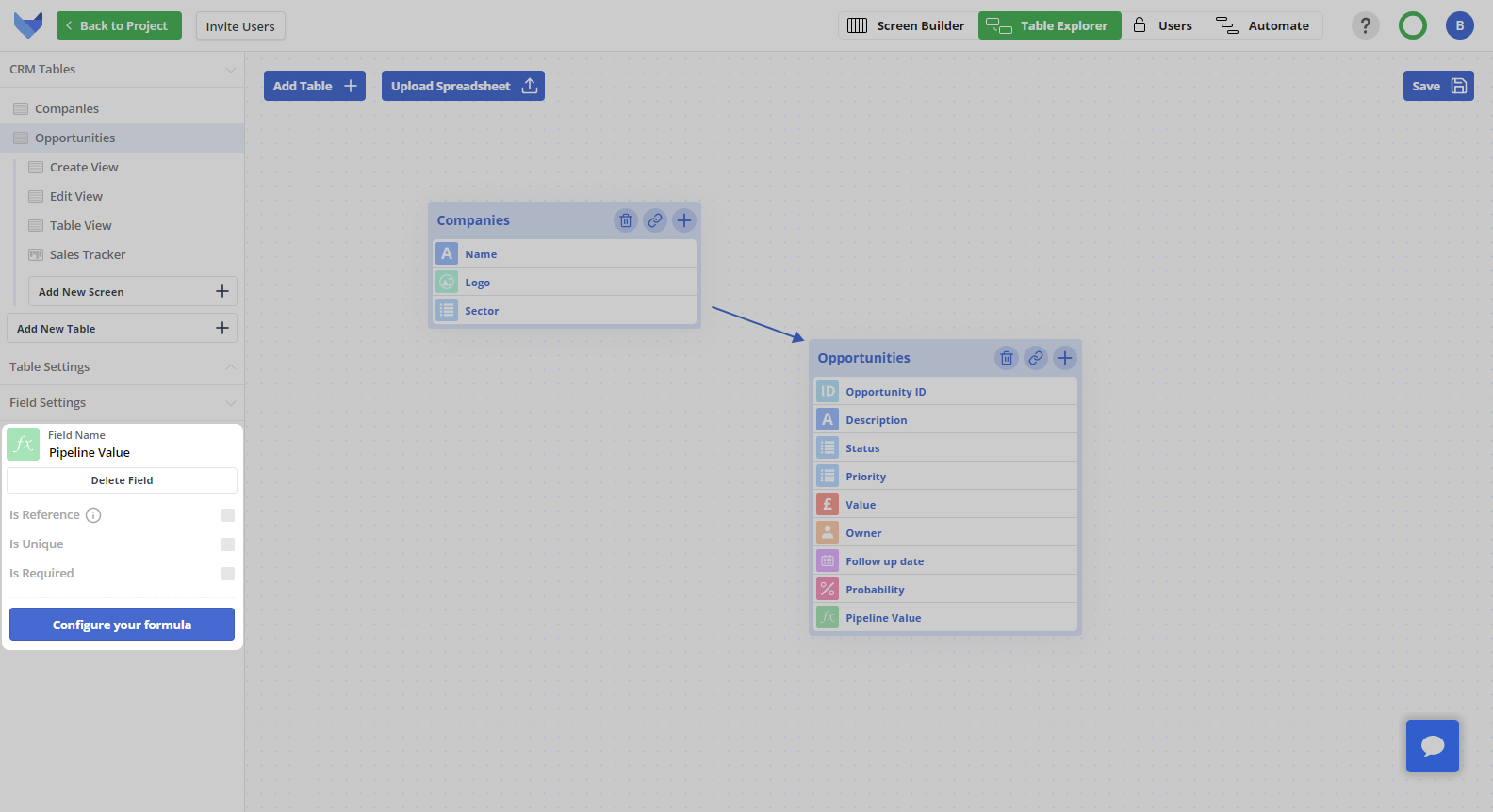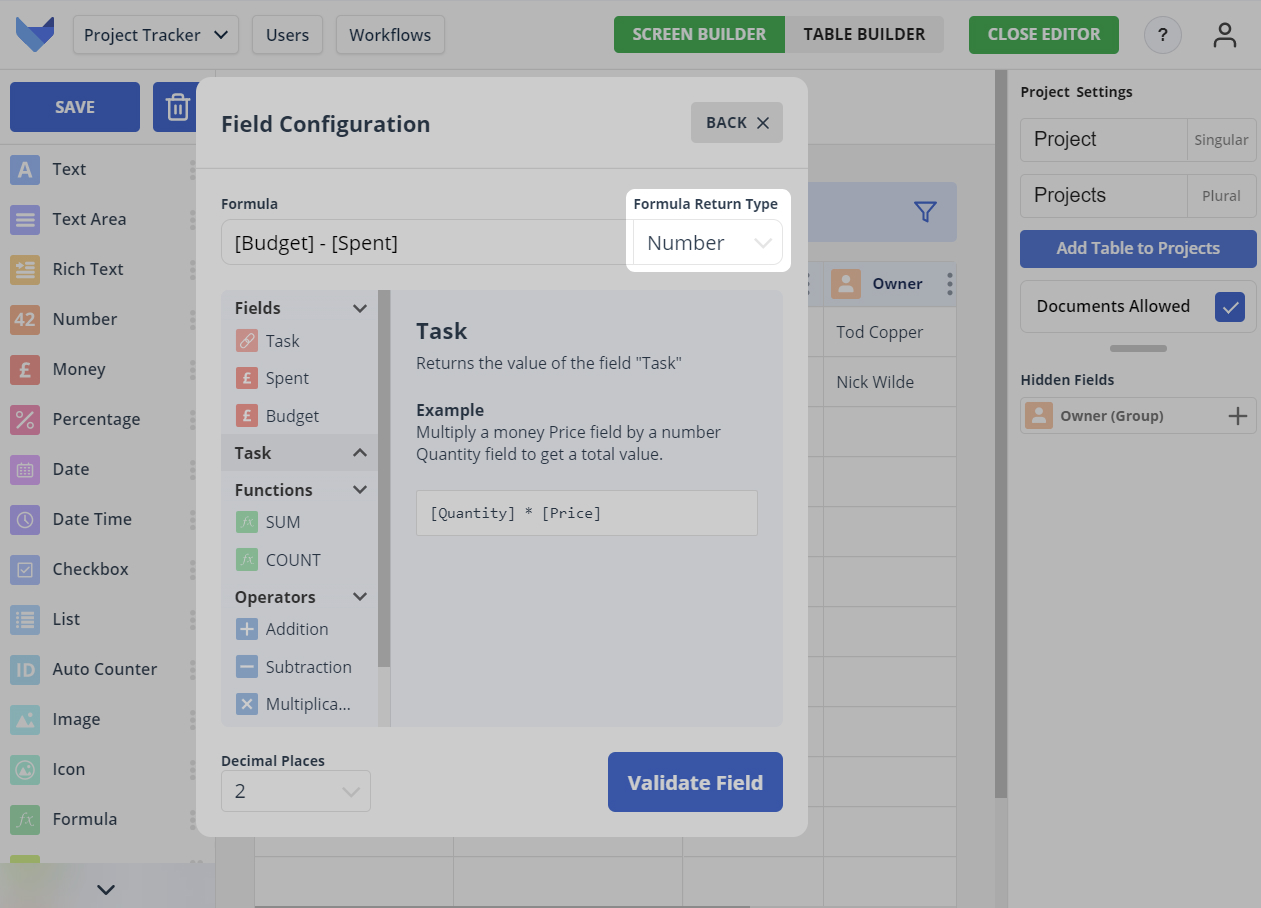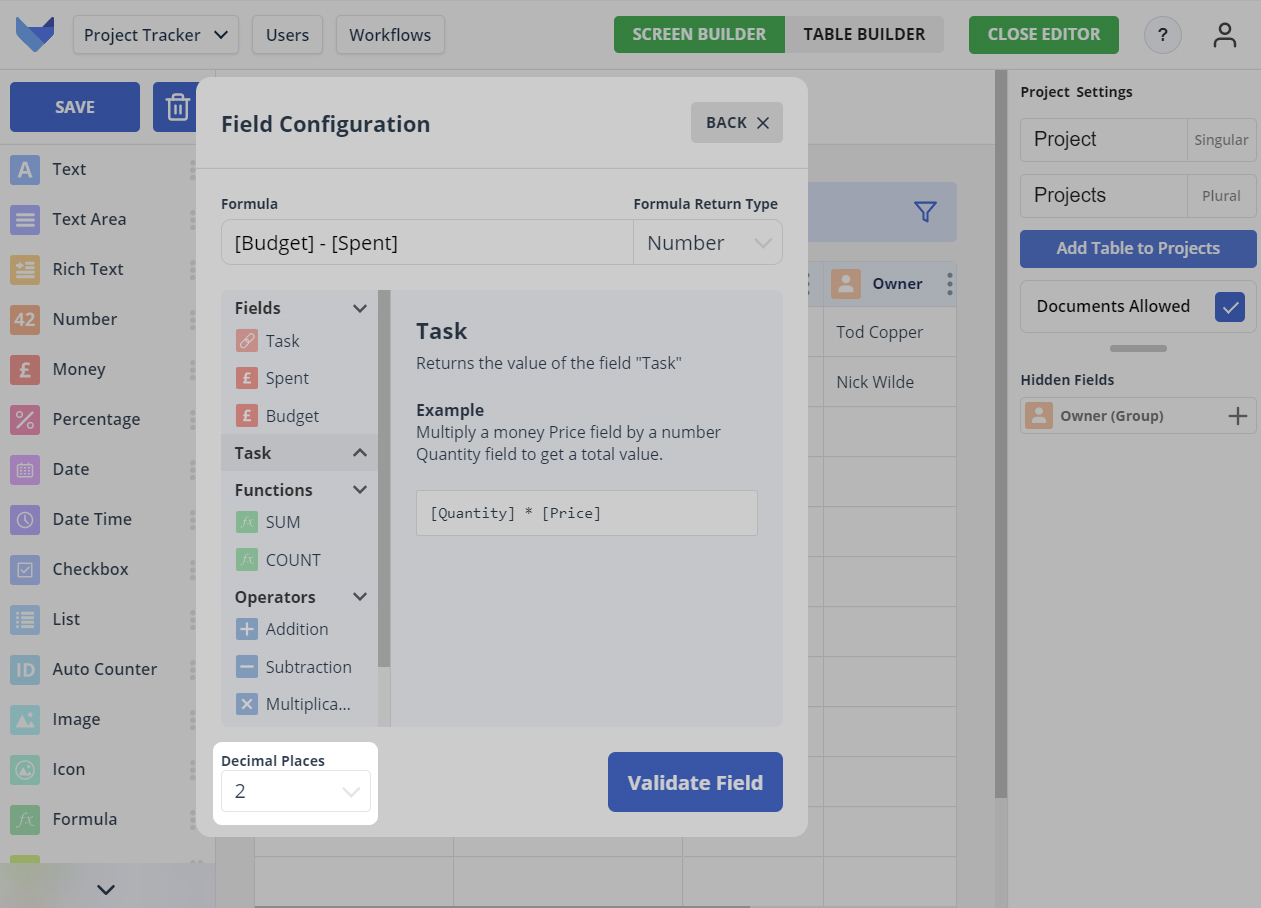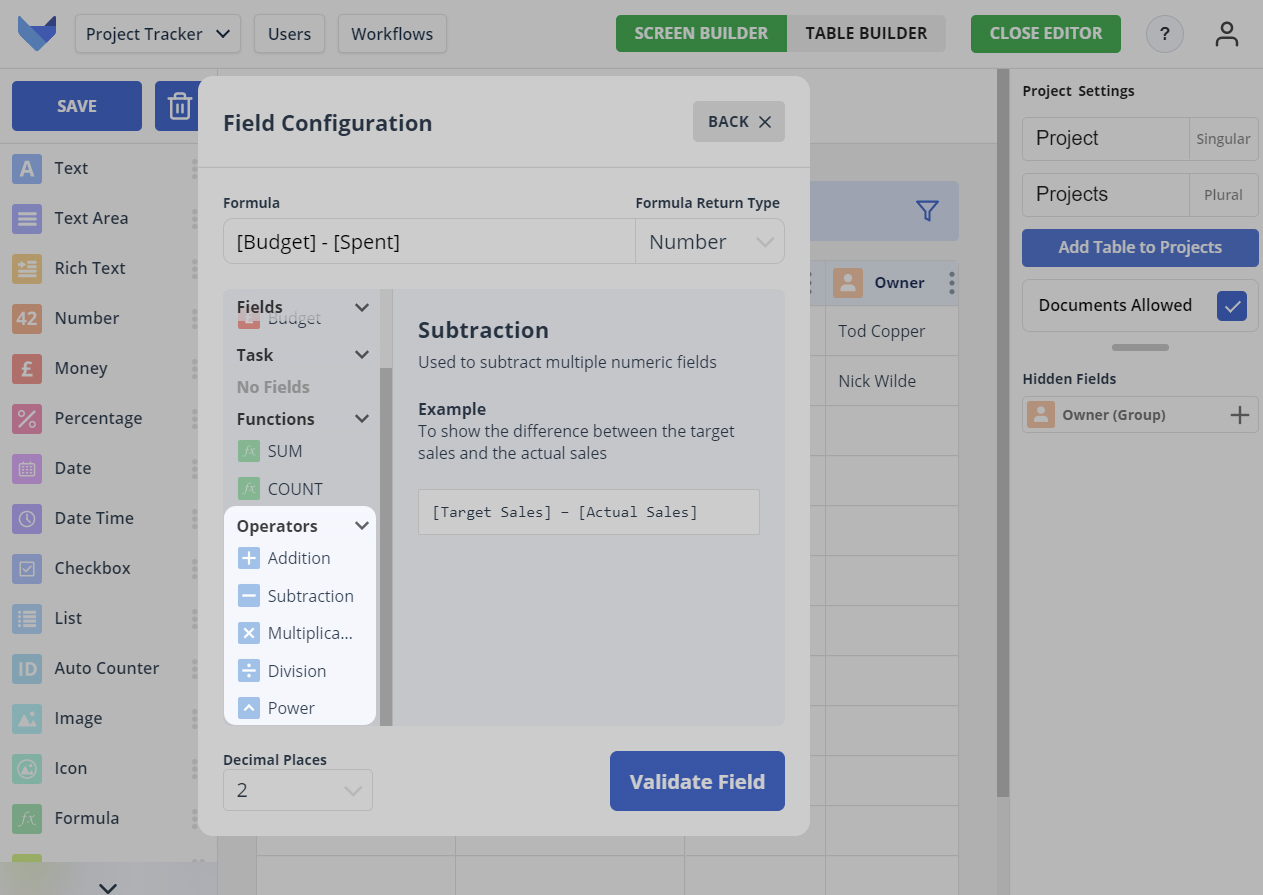# Formula Fields

Formula Fields can be used to perform calculations on data within other Fields in your Tables.

You can use basic arithmetic as well as more complex operations such as summing the total value in a Field on related child Records.

## Configuring a Formula Field

To configure the formula for a Formula Field, you will need to open the Field Settings Menu. This can be done in either the Grid View Editor or Explorer.

Click on the Field and the settings will open in the left hand side panel.Click Configure your formula to show the formula configuration options.

You can enter the required formula. You can choose to type out the formula using the appropriate syntax, or use the menu of Fields, Operators and Functions. An explanation of the available Fields, Operators and Functions is available further down this page.

Confirm the formula is valid by clicking Validate Field and then click Save to confirm the new formula.

## Formula Return Types

Each formula must have a return type specified. The return type specifies the format of the output of the formula field.

The following return types are supported:

1. Number

Formula is calculated and shown as a number

2. Money

Formula is calculated and shown to 2 decimal places as a currency value for your chosen currency, where your currency options are: GBP, USD, EUR

You can change the currency of an existing formula, but note that this will not perform any currency conversion. The value will remain unchanged.

3. Percentage

Formula is calculated and converted to a percentage value### Specifying Decimal Places

For the number and money return types, you can specify the number of decimal places that should be shown.

The value calculated using the formula will be rounded to the specified number of decimal places.## Arithmetic in Formulas

To perform arithmetic operations in your Formula Field, you can use any of the following:

• ’-‘ for subtraction
• ‘*’ for multiplication
• ’/’ for division
• ’^’ for exponentiation, i.e. to take a value to the power of another value

Standard mathematical rules are used to determine the order of arithmetic operations.

NOTE

Ensure there is a space either side of the operation e.g. ‘1 + 2’ would be considered valid, but ‘1+2’ would not.## Referencing Fields in Formulas

To include another Field in the formula, enclose the Field Name in square brackets.

For example, if you had an Order Table with a field called ‘Quantity’ and a field called ‘Price’, you could create a formula for the total value of the Order by entering `[Quantity] * [Price]`.

You can reference Fields from linked Parent Tables using the syntax ‘ParentTableSingularName.FieldName’, ensuring you enclose this within square brackets.

For example, if you had a Project Phase Table which had a parent Project Table, assuming each Phase gets a given percentage of the Project Budget, you could create a formula for the Phase Budget by entering `[Phase Percentage] * [Project.Budget]`.

## SUM and COUNT Functions

For both the SUM and the COUNT function, you can limit the Records that are included in the summing or counting by using the SUMIF or COUNTIF functions.

The SUMIF and COUNTIF functions allow you to filter Records where the conditions of a formula are met.

As an example, you might want to count all Records in a Table where a List Field matches a certain List Value.

The syntax to use is as follows:

1. For counting the number of Records if the value in a List Field matches the specified value

`COUNTIF([<Plural Child Name>], [<Singular Child Name>.<Child List Field Name>] = "<List Item Value>")`

2. For counting the number of Records if the value in a List Field does not match the specified value

`COUNTIF([<Plural Child Name>], [<Singular Child Name>.<Child List Field Name>] != "<List Item Value>")`

3. For summing the value in a Field if the value in a List Field across Records matches the specified value

`SUMIF([<Plural Child Name>], [<Singular Child Name>.<Child List Field Name>] = "<List Item Value>")`

4. For summing the value in a Field if the value in a List Field across Records does not match the specified value

`SUMIF([<Plural Child Name>], [<Singular Child Name>.<Child List Field Name>] != "<List Item Value>")`

For example, to count the number of employees who are currently active at a company, you could add a Formula Field as `COUNTIF([Employees], [Employee.Status] = "Active")`.

### Using Brackets in Formula Fields

Brackets can be used in Formula Fields to dictate the order of operations. Compare these two formulas:

• 1 + 2 * 3 = 7
• (1 + 2) * 3 = 9

The use of brackets in the second equation changes the order of operations by making sure that the calculations inside the parentheses are done first. The result of that calculation is then used in the next step.

NOTE

Appropriate mathematical symbols should be added to validate formula operations. In the above example, (1+2)*3 would be considered valid whereas(1+2)3 would not.

## Calculating Formula Fields

Formula values are calculated just as Records are viewed. For this reason, they will always show the latest information.

NOTE

Blank values in Fields are treated as 0 for formula fields.